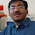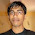### Shortest Distance from NewYork to Mumbai

Source: Discussions with BX Mumbai team on my flight from New York to Mumbai

Problem: The flight from New York (Newark - EWR) to Mumbai (Bombay - BOM) takes the aerial route as shown in the figure. Why do the flights not take straightline distance to minimize cost?

Hint: The answer is mathematical. Do not think of regulatory or political reasons.

Update: (19-07-2012) Assume earth is perfect sphere

1.Daya!!

http://en.wikipedia.org/wiki/Great_circle

2.I can think of multiple reasons like radar visibility, refueling etc but as the answer needs to be mathematical i will go with this one. The straight line path is not the shortest path as the earth is flatter at the equator. The arc through the regions close to the poles is the shorter path.

3.Probably because that IS the shortest distance with earth being elliptical... and that it goes from over the arctic circle...

4.Acutually it is a straight line. It just doesn't looks like one because you are looking to a planar representation of an sphere (the earth).

5.The way I see it, they are indeed flying a straight line.

6.Let the start points be 's' and the end point be 'e'. For a spherical surface, the shortest path is given by the intersection of the plane formed by origin,s & e and the surface of the sphere. I don't remember the exact term, but I think its called the "Geodesic Path".

For other economical reasons( refuelling etc. ), instead of following this path, they fly via London.

7.The shortest distance between two points on a sphere is the one which lies on great circles. Great circle is the circle on sphere which has same center. However when you put it on the 2D map it looks curved. In short shortest distance will be through the earth however on surface of earth its a curved path.

8.Is it the geodesic?

9.cause path is projection of spherical straight line into 2d where it looks a curve.

10.Jus making a hunch here. I think they must be taking the smallest path flying over the great circle joining the two points. On flattened maps however, owing to the distortion the path appears curved.

11.Geodesic? Although I thought physical considerations are also involved(e.g. jet streams) that increase the mileage.

12.Because Thomas Friedman not withstanding, the world is not flat.

13.Is it because the earth is round and the map is flat? I am not sure. But eagerly waiting to know the correct answer and also if my understanding is correct.

14.The Shortest path between any two points is the path taken by the light to go from one end to the other. Now, the similar concept applies to the flight of the aeroplanes. The speed of these planes are not the same at all points but varies with the density of air etc. So,any aeroplane takes that path depending on its varying speed (simialr to refractive index of light)

15.The principle is similar to the refractive index for light. Had the light's refractive index(say speed) been same in all mediums, it would have always travelled in a straight line path.But this is not the case. With varying refractive index, it has to accordingly vary its path so that the time taken to reach between any two end points is still the minimum.
Same applies to the aeroplane. the speed varies for the aeroplane depending on many factors and with varying speed, it adjusts its path. Initially at lower heights as it moves up, it bends towards the normal and vice versa.

16.they are taking the straight line distance. on a sphere.
plotted on a 2D surface, it doesn't look straight anymore.

17.They follow geodesics on the earth (treated as a sphere). On the earth, the geodesic would be the (unique) great circle passing through the two points. Although some other regulatory reasons might also affect flight paths, the one in question (EWR-BOM) is quote close to the geodesic.

18.This is a sphere flattened on a map. The route if plotted on a globe would be the shortest path.

19.I guess the reason is to get the flight into the path of one of the jet streams. Either the tropical jet or the polar jet.

Jet streams are fast flowing, narrow air currents found in the atmospheres of some planets, including Earth.
The location of the jet stream is extremely important for aviation.
This saves on the the flight time and hence the fuel cost.

Details of the jet streams can be found at ..

http://en.wikipedia.org/wiki/Jet_stream

20.Yes, it is a straight line.
http://en.wikipedia.org/wiki/Geodesic

21.@shantanu, gujju, uttam, ankush jain, siva, asad, nishant totla (infinity).. correcto! :)

@johnny, Unknown.. assume earth is round.. will add this assumption in the question. thanks